# C++ Programs to Insert Element in Array

In this tutorial you will learn about the C++ Programs to Insert Element in Array and its application with practical example.

## C++ Program to Insert Element in Array

In this tutorial, we will learn to create a C++ program that will do Insert Element in Array using C++ programming.

## Prerequisites

Before starting with this tutorial we assume that you are best aware of the following C++ programming topics:

• Operators in C++ Programming.
• Basic Input and Output function in C++ Programming.
• Basic C++ programming.
• For loop in C++ Programming.
• Creating and Using the user-defined function in C++ programming.

## Insert Element in Array:-

In this tutorial, we will make a program that will insert the element in the array using c++ programming. First will take the five elements of an array from the user. Then will take the new elements from the user for inserting in the array. And at last, we will insert that element at the end of the array.

## Output:-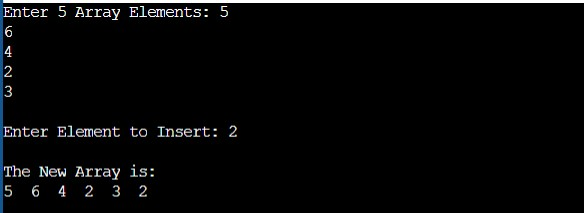In the above program, we have first declared and initialized a set of variables required in the program.

• i = it will hold the integer value.
• arr = it will hold the elements in an array.
• elem = it will hold the integer value that will be inserted in the array.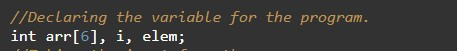Taking input from the user:-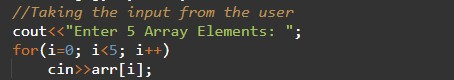New elements from the user.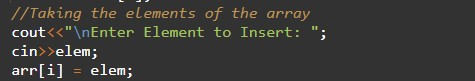Taking input elements to add from the user.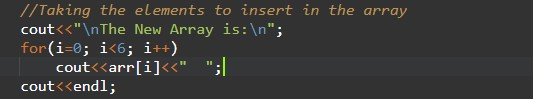In this tutorial we have learn about the C++ Programs to Insert Element in Array and its application with practical example. I hope you will like this tutorial.